Line Chart using Plotly in Python

• Last Updated : 12 Feb, 2021

Plotly is a Python library which is used to design graphs, especially interactive graphs. It can plot various graphs and charts like histogram, barplot, boxplot, spreadplot and many more. It is mainly used in data analysis as well as financial analysis. plotly is an interactive visualization library.

Line Chart in Plotly

Line plot in Plotly is much accessible and illustrious annexation to plotly which manage a variety of types of data and assemble easy-to-style statistic. With px.line each data position is represented as a vertex transformed(which location is given by the x and y columns) of a polyline mark in 2D space. Line chart Displays a series of numerical data as points which are connected by lines. It visualizes to show two data trends. The main productive feature is it can display thousands of data points without scrolling. It can be created using the line() method of plotly.express class.

Syntax: plotly.express.line(data_frame=None, x=None, y=None, line_group=None, color=None, line_dash=None, hover_name=None, hover_data=None, custom_data=None, text=None, facet_row=None, facet_col=None, facet_col_wrap=0, error_x=None, error_x_minus=None, error_y=None, error_y_minus=None, animation_frame=None, animation_group=None, category_orders={}, labels={}, orientation=None, color_discrete_sequence=None, color_discrete_map={}, line_dash_sequence=None, line_dash_map={}, log_x=False, log_y=False, range_x=None, range_y=None, line_shape=None, render_mode=’auto’, title=None, template=None, width=None, height=None)

Parameters:

Creating a simple line chart

Example 1: In this example, we will see a simple line plot with two different datasets.

Python3

 import plotly.express as px   x = [1,2,3,4,5] y = [1,3,4,5,6] fig = px.line( x = x ,               y = y,               title = 'A simple line graph') fig.show()

Output: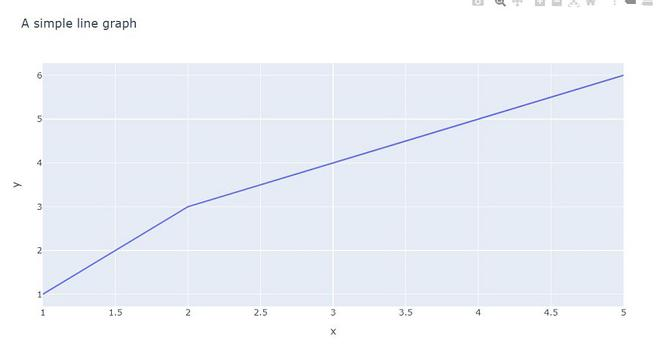Example 2: In this example we will plot using go.Scatter. plotly.express has two functions scatter and line, go.Scatter can be used both for plotting points (makers) or lines, depending on the value of mode.

Python3

 import numpy as np import plotly.graph_objects as go   x = [0, 1, 2, 3, 4, 5, 6, 7, 8, 9] y = [1, 3, 4, 5, 6] fig = go.Figure(data=go.Scatter(x = x, y = y)) fig.show()

Output: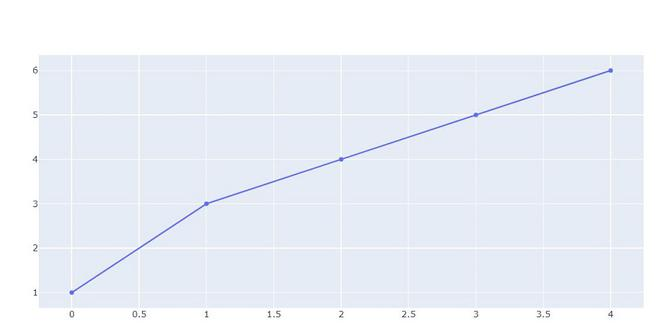Example 3: Using the Iris dataset we will plot the line chart.

Python3

 import plotly.express as px   # Loading the iris dataset df = px.data.iris()   fig = px.line(df, x="sepal_width", y="sepal_length") fig.show()

Output: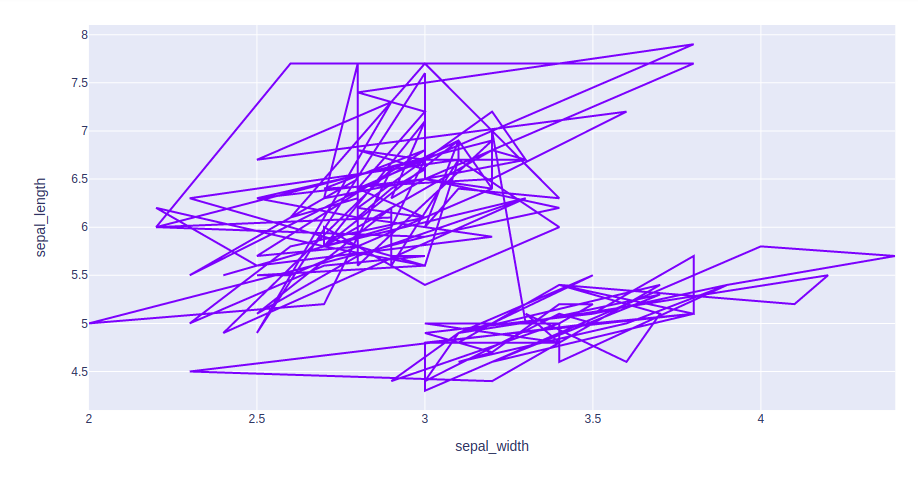Mapping the encoding color

Column encoding color is also known as, color mapping which is a type of method which represents text or numeric data in colors inline form.

Example 1: In This example, we will use color attributes to plot the line with a different color.

Python3

 import plotly.express as px   df = px.data.iris().head(20)   fig = px.line(df, x = "sepal_width",               y = "sepal_length" ,               color = "sepal_length") fig.show()

Output: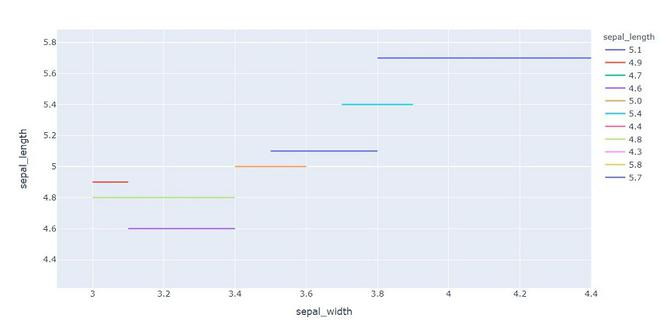Example 2: In this example, we will use different color aspect.

Python3

 import plotly.express as px   # Loading the iris dataset df = px.data.iris()   fig = px.line(df, x = "sepal_width", y = "sepal_length",               color = "species") fig.show()

Output: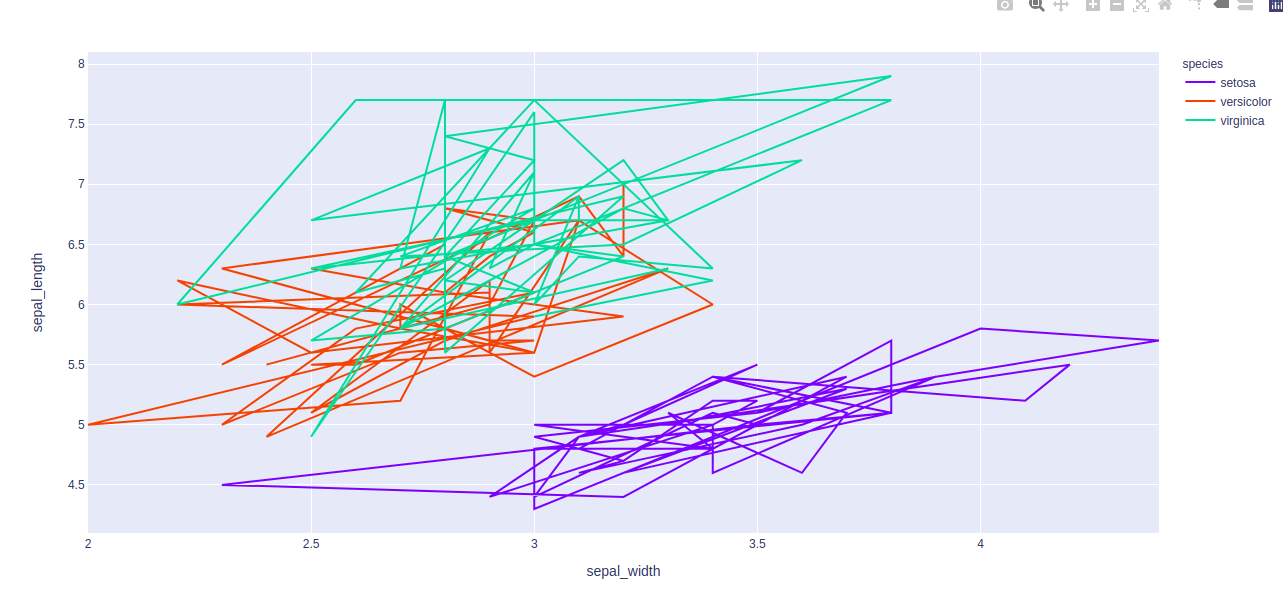My Personal Notes arrow_drop_up
Recommended Articles
Page :